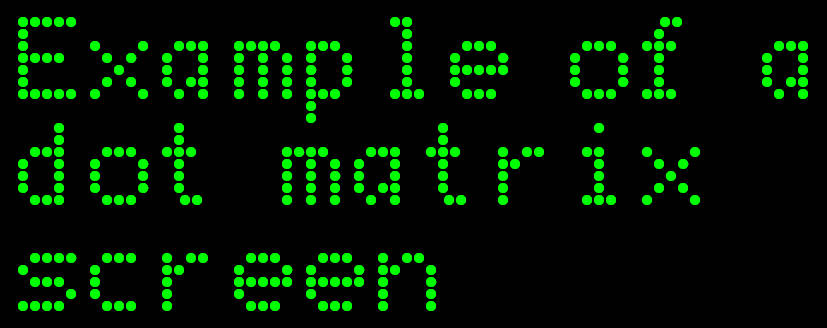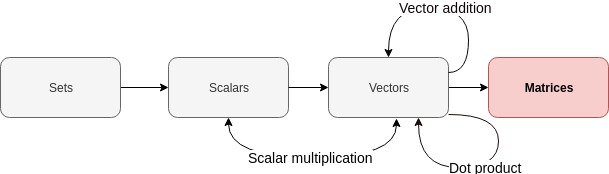Want to share your content on R-bloggers? click here if you have a blog, or here if you don't.

(TL;DR: matrices are rectangular arrays of numbers.)

LaTeX and MathJax warning for those viewing my feed: please view
directly on website!I wanted to avoid a picture related to the film franchise!

Me

Last
time
,
we learnt about dot products. We will now finally start talking
aobut matrices.# Today’s topic: Matrices – a primer

We know what row vectors and column vectors are from our previous posts.

What would it look like if we were to stack a bunch of row vectors on
top of each other? Similarly, what would it look like if we were to put a
bunch of column vectors next to each other? Let’s experiment!

Let’s define some row vectors:

Let’s stack them on top of each other:

This rectangular array of numbers is an example of a matrix!

Let’s repeat the exercise with the same vectors represented as column
vectors:

Putting these column vectors next to each other gives us this:

Looks like another matrix to me!

In fact, our row vectors and column vectors we saw in previous posts
live double lives. A row vector goes by another name: “row matrix”.
A column vector goes by another name, too: “column matrix”.

Sneaky!

# A little bit of notation

As before, we will be following the notation used in Goodfellow, Ian,
et al.
. We will
depict matrices using uppercase, bold font:

# Describing the size of a matrix

From the above, we can guess that matrices can be described in terms of
rows and columns. How can we describe the number of rows and the
number of columns
we have in our matrices?

Let’s go back to our previous examples. They both happen to have three
rows
and three columns. So we have ourselves two 3 x 3 (“three by
three”) matrices
. The number of rows and the number of columns
describe the “size” of our matrix.

Let’s generalise this. By convention, we have $m$ rows and $n$ columns
in our matrix. Let’s use our matrix $\boldsymbol{A}$ as an example. We
haven’t defined what elements it contains. In fact, all we have said is
that $\boldsymbol{A}$ is a matrix. Whatever the contents of
$\boldsymbol{A}$, it will will have $m$ rows and $n$ columns. So
$\boldsymbol{A}$ will be of size $m \times n$.

# Keep it real

Let’s take this a step further. From previous posts, we are working with
real numbers so let’s assume that our matrix $\boldsymbol{A}$ of
size $m \times n$ will contain real numbers. That is, it is a real
matrix
. How can we depict this using some compact notation?

Remember when we touched on our vectors belonging to some abstract
set of all real-valued vectors of similar dimensions when describing the dimensions of a
vector
?
We can say something similar for our real-valued matrix of size
$m \times n$. We can describe this set of all $m \times n$ real-valued matrices like this:

So to show that our matrix $\boldsymbol{A}$ belongs to the set of all
$m \times n$ matrices that are made up of real numbers, we simply say:

Going back to our examples above:

• Our real-valued row vectors (a.k.a. row matrices) are members of
the set $\mathbb{R}^{1 \times n}$. That is, they are members of the
set of all possible matrices made up of one row and $n$ columns of
real numbers.
• Our real-valued column vectors (a.k.a. column matrices) are
members of the set $\mathbb{R}^{m \times 1}$. That is, they are
members of the set of all possible matrices made up of $m$ rows and one column of real numbers.
• Our real-valued square matrices are members of the set
$\mathbb{R}^{m \times n}$ where $m == n$. That is, they are members
of the set of all possible real matrices that have the same number
of rows as they have columns.

# How can we create matrices in R?

## Stack those vectors

One way is to simply rbind and cbind vectors. Let’s define 3 vectors
and rbind them (i.e. stack them row-wise):

a <- c(1, 2, 3)
b <- c(4, 5, 6)
c <- c(7, 8, 9)

A <- rbind(a, b, c)

print(A)

##   [,1] [,2] [,3]
## a    1    2    3
## b    4    5    6
## c    7    8    9


We have ourselves a matrix!

print(class(A))

##  "matrix"


Let’s now stack them column-wise by using cbind on our vectors:

A <- cbind(a, b, c)

print(A)

##      a b c
## [1,] 1 4 7
## [2,] 2 5 8
## [3,] 3 6 9


It’s a matrix!

print(class(A))

##  "matrix"


## Use the matrix function

The “usual” way to create a matrix would be to pass some vector or list
into the matrix function and specify its dimensions. For example, if we
want to use the values passed into the matrix function to create a 3×3 matrix by using the values in a column-wise manner, we can do this:

matrix(1:9, nrow=3, ncol=3)

##      [,1] [,2] [,3]
## [1,]    1    4    7
## [2,]    2    5    8
## [3,]    3    6    9


If instead we want to use the numbers in a row-wise manner, then we
can use the byrow argument and set it to TRUE:

matrix(1:9, nrow=3, ncol=3, byrow=TRUE)

##      [,1] [,2] [,3]
## [1,]    1    2    3
## [2,]    4    5    6
## [3,]    7    8    9


The data types of the elements in the vector or list you pass into the
matrix function will be coerced to a single data type following the
usual coercion rules. For example, if we use a vector containing a
numeric type and a integer type, let’s see what happens:

our_vec <- c(1.0, 1L)
A <- matrix(our_vec, nrow=2, ncol=1)

print(A)

##      [,1]
## [1,]    1
## [2,]    1


From previous posts, we know that the values in our vector have already
been coerced by c before the matrix function sees them! So,
naturally, we have a matrix of numeric types:

sapply(A, class)

##  "numeric" "numeric"


We can even have a character matrix. Not sure why we’d want to create
one…but we can!

x <- c("hello", "goodbye")
matrix(x)

##      [,1]
## [1,] "hello"
## [2,] "goodbye"


## Elements are recycled

Recycling occurs like in vector addition posts. For example, if we try to
create a matrix with four elements (2 rows times 2 columns) from a
vector with only two elements, this is what we get:

matrix(c(1, 2), nrow=2, ncol=2)

##      [,1] [,2]
## [1,]    1    1
## [2,]    2    2


If the number of elements in our vector is not a multiple or submultiple
of the number of rows or columns, we get warned of this fact:

matrix(c(1, 2, 3), nrow=2, ncol=1)

## Warning in matrix(c(1, 2, 3), nrow = 2, ncol = 1): data length  is not a
## sub-multiple or multiple of the number of rows 

##      [,1]
## [1,]    1
## [2,]    2


Done!

# Conclusion

Next time, we’ll talk about matrix addition and introduce some notation we can use to access the individual elements contained within our matrices!

Justin